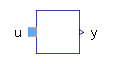Magnetic Flux Sensor - MapleSim Help

Magnetic Flux Sensor

Sensor to measure magnetic fluxDescription The Magnetic Flux Sensor component generates a signal output proportional to the magnetic flux through it.Equations $\mathrm{\Phi }={\mathrm{\Phi }}_{p}=-{\mathrm{\Phi }}_{n}$ ${V}_{{m}_{p}}={V}_{{m}_{n}}$Connections

 Name Description Modelica ID ${\mathrm{port}}_{p}$ Positive magnetic port port_p ${\mathrm{port}}_{n}$ Negative magnetic port port_n $\mathrm{\Phi }$ Magnetic flux from port p to port n as output signal PhiModelica Standard Library The component described in this topic is from the Modelica Standard Library. To view the original documentation, which includes author and copyright information, click here.